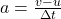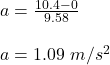## Usain Bolt, an Olympian from Jamaica, is the fastest human ever recorded. Determine his average rate of acceleration if he achieved a speed

Question

Usain Bolt, an Olympian from Jamaica, is the fastest human ever recorded. Determine his average rate of acceleration if he achieved a speed of 10.4 m/s in 9.58 sec. (Think about what a sprinter’s initial speed is.)

in progress 0
2 months 2021-07-21T12:37:51+00:00 2 Answers 1 views 0

1.09 m/s²

Explanation:

Given:

Final speed of the sprinter (v) = 10.4 m/s

Initial speed of the sprinter (u) = 0 m/s (As the sprinter starts from rest position)

Time taken to reach the speed(Δt) = 9.58 s

Average acceleration is defined as the rate of change of velocity.

So, the acceleration of the sprinter is given as:Plug in all the given values and solve for acceleration, ‘a’. This gives,Therefore, the magnitude of the average acceleration of the sprinter is 1.09 m/s².